Publicité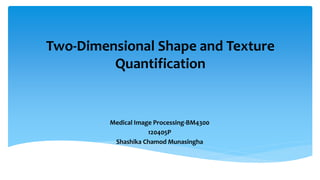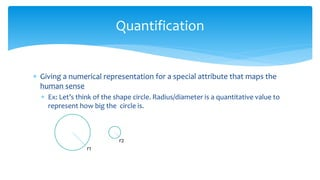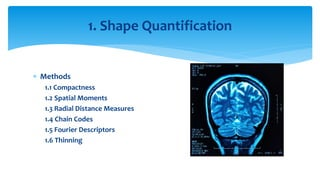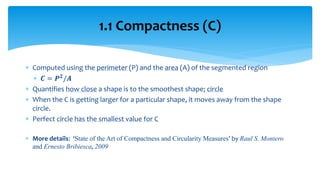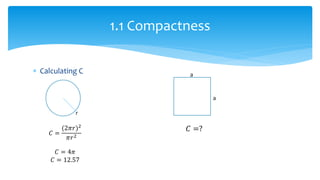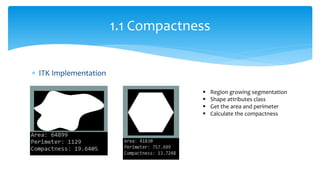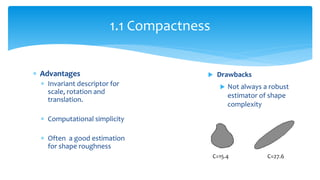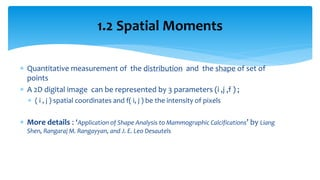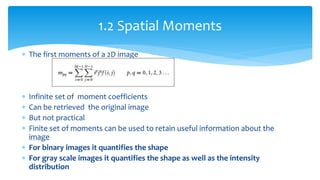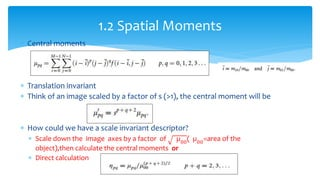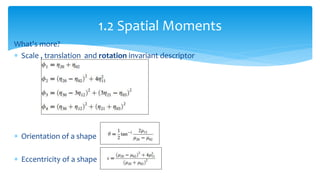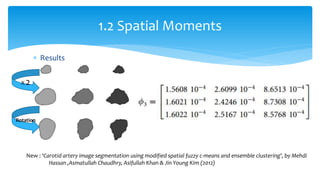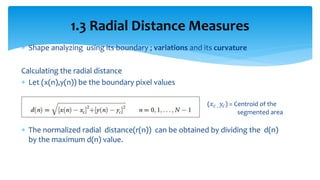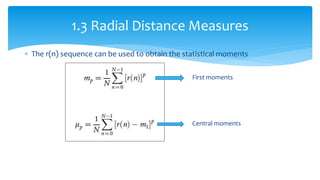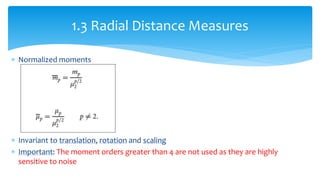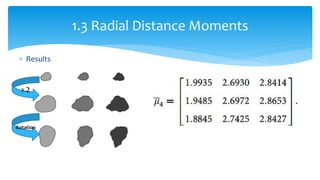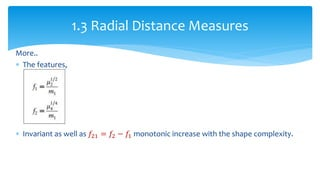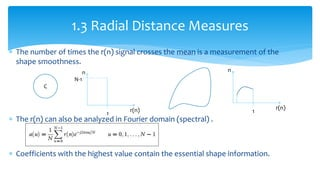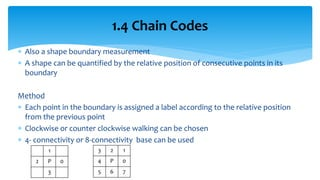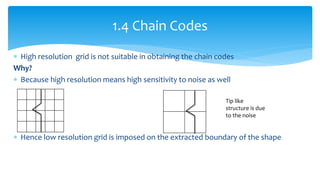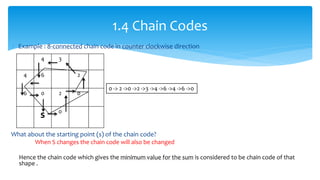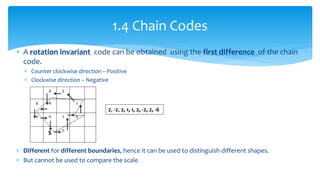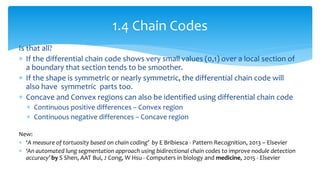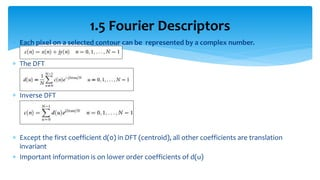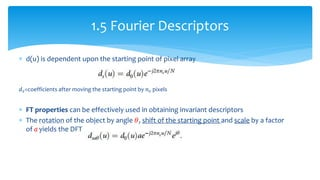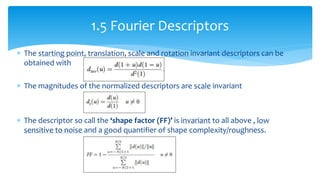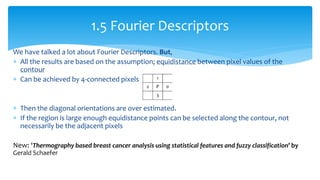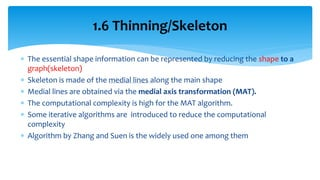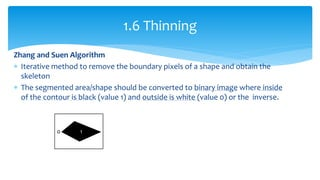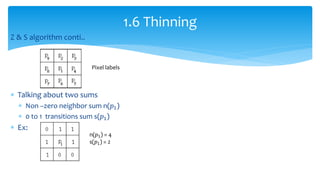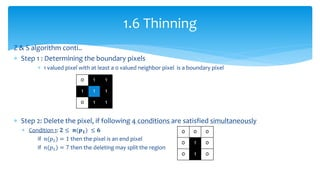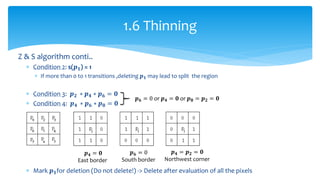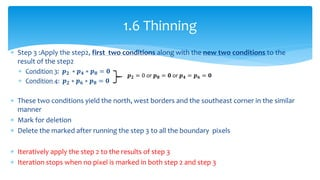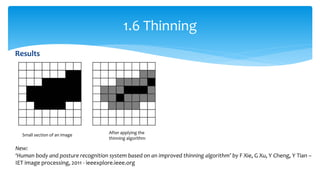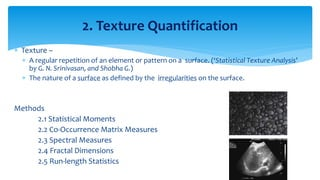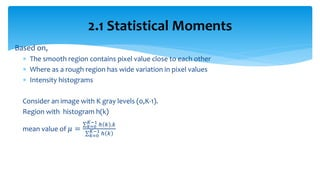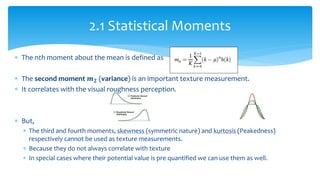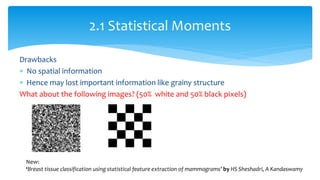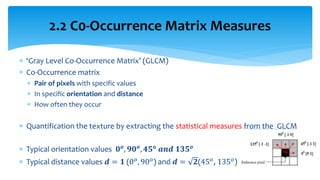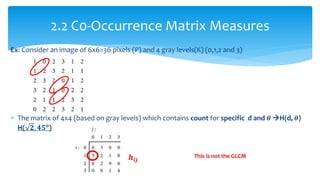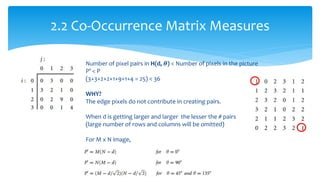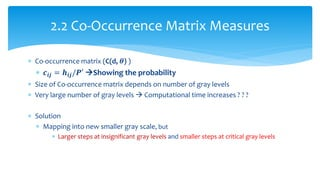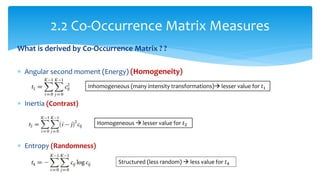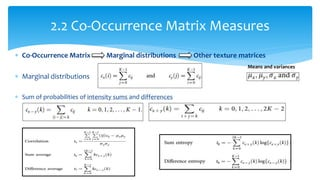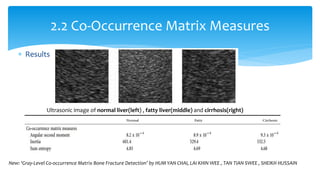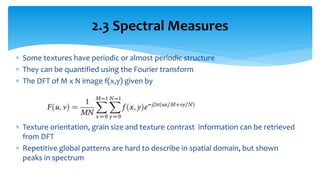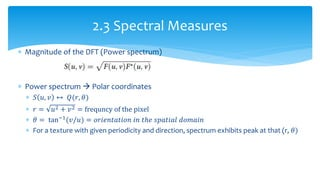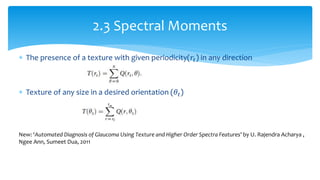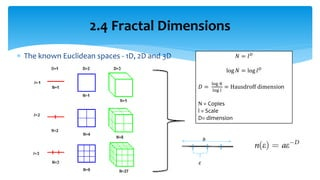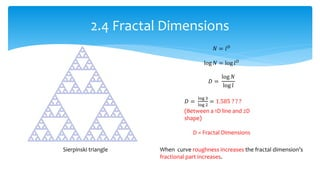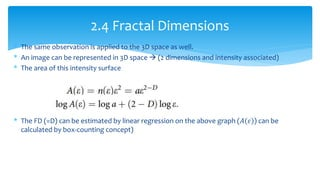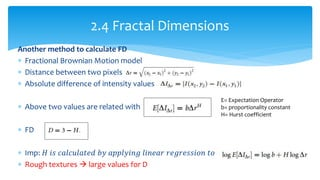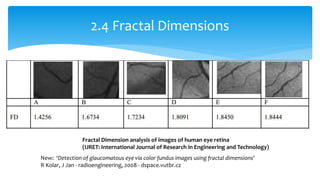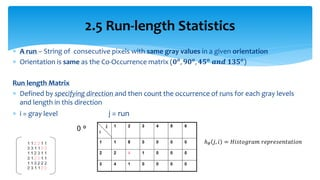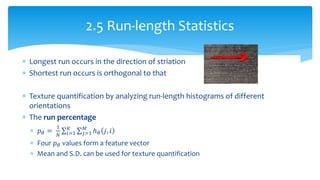1 sur 57
Publicité

### Two Dimensional Shape and Texture Quantification - Medical Image Processing

1. Two-Dimensional Shape and Texture Quantification Medical Image Processing-BM4300 120405P Shashika Chamod Munasingha
2.  Giving a numerical representation for a special attribute that maps the human sense  Ex: Let’s think of the shape circle. Radius/diameter is a quantitative value to represent how big the circle is. Quantification r1 r2
3.  Methods 1.1 Compactness 1.2 Spatial Moments 1.3 Radial Distance Measures 1.4 Chain Codes 1.5 Fourier Descriptors 1.6 Thinning 1. Shape Quantification
4.  Computed using the perimeter (P) and the area (A) of the segmented region  𝑪 = 𝑷 𝟐/𝑨  Quantifies how close a shape is to the smoothest shape; circle  When the C is getting larger for a particular shape, it moves away from the shape circle.  Perfect circle has the smallest value for C  More details: ‘State of the Art of Compactness and Circularity Measures’ by Raul S. Montero and Ernesto Bribiesca, 2009 1.1 Compactness (C)
5.  Calculating C 1.1 Compactness 𝐶 = (2𝜋𝑟)2 𝜋𝑟2 𝐶 = 4𝜋 𝐶 = 12.57 r a a 𝐶 =?
6.  ITK Implementation 1.1 Compactness  Region growing segmentation  Shape attributes class  Get the area and perimeter  Calculate the compactness
7.  Advantages  Invariant descriptor for scale, rotation and translation.  Computational simplicity  Often a good estimation for shape roughness 1.1 Compactness  Drawbacks  Not always a robust estimator of shape complexity C=15.4 C=27.6
8.  Quantitative measurement of the distribution and the shape of set of points  A 2D digital image can be represented by 3 parameters (i ,j ,f ) ;  ( i , j ) spatial coordinates and f( i, j ) be the intensity of pixels  More details : ‘Application of Shape Analysis to Mammographic Calcifications’ by Liang Shen, Rangaraj M. Rangayyan, and J. E. Leo Desautels 1.2 Spatial Moments
9.  The first moments of a 2D image  Infinite set of moment coefficients  Can be retrieved the original image  But not practical  Finite set of moments can be used to retain useful information about the image  For binary images it quantifies the shape  For gray scale images it quantifies the shape as well as the intensity distribution 1.2 Spatial Moments
10.  Central moments  Translation invariant  Think of an image scaled by a factor of s (>1), the central moment will be  How could we have a scale invariant descriptor?  Scale down the image axes by a factor of µ00 ( µ00 =area of the object),then calculate the central moments or  Direct calculation 1.2 Spatial Moments
11. What's more?  Scale , translation and rotation invariant descriptor  Orientation of a shape  Eccentricity of a shape 1.2 Spatial Moments
12.  Results 1.2 Spatial Moments X 2 Rotation New : ‘Carotid artery image segmentation using modified spatial fuzzy c-means and ensemble clustering’, by Mehdi Hassan ,Asmatullah Chaudhry, Asifullah Khan & Jin Young Kim (2012)
13.  Shape analyzing using its boundary ; variations and its curvature Calculating the radial distance  Let (x(n),y(n)) be the boundary pixel values  (𝑥 𝑐 , 𝑦𝑐) = Centroid of the segmented area  The normalized radial distance(r(n)) can be obtained by dividing the d(n) by the maximum d(n) value. 1.3 Radial Distance Measures
14.  The r(n) sequence can be used to obtain the statistical moments First moments Central moments 1.3 Radial Distance Measures
15.  Normalized moments  Invariant to translation, rotation and scaling  Important: The moment orders greater than 4 are not used as they are highly sensitive to noise 1.3 Radial Distance Measures
16.  Results 1.3 Radial Distance Moments X 2 Rotation
17. More..  The features,  Invariant as well as 𝑓21 = 𝑓2 − 𝑓1 monotonic increase with the shape complexity. 1.3 Radial Distance Measures
18.  The number of times the r(n) signal crosses the mean is a measurement of the shape smoothness.  The r(n) can also be analyzed in Fourier domain (spectral) .  Coefficients with the highest value contain the essential shape information. 1.3 Radial Distance Measures C r(n) 1 N-1 n r(n) 1 n
19.  Also a shape boundary measurement  A shape can be quantified by the relative position of consecutive points in its boundary Method  Each point in the boundary is assigned a label according to the relative position from the previous point  Clockwise or counter clockwise walking can be chosen  4- connectivity or 8-connectivity base can be used 1.4 Chain Codes 3 2 1 4 P 0 5 6 7 1 2 P 0 3
20.  High resolution grid is not suitable in obtaining the chain codes Why?  Because high resolution means high sensitivity to noise as well  Hence low resolution grid is imposed on the extracted boundary of the shape 1.4 Chain Codes Tip like structure is due to the noise
21.  Example : 8-connected chain code in counter clockwise direction What about the starting point (s) of the chain code? When S changes the chain code will also be changed Hence the chain code which gives the minimum value for the sum is considered to be chain code of that shape . 1.4 Chain Codes 4 3 4 6 2 6 0 2 0 s 0 0 -> 2 ->0 ->2 ->3 ->4 ->6 ->4 ->6 ->0
22.  A rotation invariant code can be obtained using the first difference of the chain code.  Counter clockwise direction – Positive  Clockwise direction – Negative  Different for different boundaries, hence it can be used to distinguish different shapes.  But cannot be used to compare the scale 1.4 Chain Codes 2, -2, 2, 1, 1, 2, -2, 2, -6
23. Is that all?  If the differential chain code shows very small values (0,1) over a local section of a boundary that section tends to be smoother.  If the shape is symmetric or nearly symmetric, the differential chain code will also have symmetric parts too.  Concave and Convex regions can also be identified using differential chain code  Continuous positive differences – Convex region  Continuous negative differences – Concave region New:  ‘A measure of tortuosity based on chain coding’ by E Bribiesca - Pattern Recognition, 2013 – Elsevier  ‘An automated lung segmentation approach using bidirectional chain codes to improve nodule detection accuracy’ by S Shen, AAT Bui, J Cong, W Hsu - Computers in biology and medicine, 2015 - Elsevier 1.4 Chain Codes
24.  Each pixel on a selected contour can be represented by a complex number.  The DFT  Inverse DFT  Except the first coefficient d(0) in DFT (centroid), all other coefficients are translation invariant  Important information is on lower order coefficients of d(u) 1.5 Fourier Descriptors
25.  d(u) is dependent upon the starting point of pixel array 𝑑 𝑠=coefficients after moving the starting point by 𝑛 𝑠 pixels  FT properties can be effectively used in obtaining invariant descriptors  The rotation of the object by angle 𝜃, shift of the starting point and scale by a factor of a yields the DFT series 1.5 Fourier Descriptors
26.  The starting point, translation, scale and rotation invariant descriptors can be obtained with  The magnitudes of the normalized descriptors are scale invariant  The descriptor so call the ‘shape factor (FF)’ is invariant to all above , low sensitive to noise and a good quantifier of shape complexity/roughness. 1.5 Fourier Descriptors
27. We have talked a lot about Fourier Descriptors. But,  All the results are based on the assumption; equidistance between pixel values of the contour  Can be achieved by 4-connected pixels  Then the diagonal orientations are over estimated.  If the region is large enough equidistance points can be selected along the contour, not necessarily be the adjacent pixels New: ‘Thermography based breast cancer analysis using statistical features and fuzzy classification’ by Gerald Schaefer 1.5 Fourier Descriptors
28.  The essential shape information can be represented by reducing the shape to a graph(skeleton)  Skeleton is made of the medial lines along the main shape  Medial lines are obtained via the medial axis transformation (MAT).  The computational complexity is high for the MAT algorithm.  Some iterative algorithms are introduced to reduce the computational complexity  Algorithm by Zhang and Suen is the widely used one among them 1.6 Thinning/Skeleton
29. Zhang and Suen Algorithm  Iterative method to remove the boundary pixels of a shape and obtain the skeleton  The segmented area/shape should be converted to binary image where inside of the contour is black (value 1) and outside is white (value 0) or the inverse. 1.6 Thinning 0 1
30. Z & S algorithm conti..  Talking about two sums  Non –zero neighbor sum n(𝑝1)  0 to 1 transitions sum s(𝑝1)  Ex: 1.6 Thinning n(𝑝1) = 4 s(𝑝1) = 2 Pixel labels
31. Z & S algorithm conti..  Step 1 : Determining the boundary pixels  1 valued pixel with at least a 0 valued neighbor pixel is a boundary pixel  Step 2: Delete the pixel, if following 4 conditions are satisfied simultaneously  Condition 1: 𝟐 ≤ 𝒏(𝒑 𝟏) ≤ 𝟔 if 𝑛(𝑝1) = 1 then the pixel is an end pixel if 𝑛(𝑝1) = 7 then the deleting may split the region 1.6 Thinning 0 1 1 1 1 1 0 1 1 0 0 0 0 1 0 0 1 0
32. Z & S algorithm conti..  Condition 2: s(𝒑 𝟏) = 1  If more than 0 to 1 transitions ,deleting 𝒑 𝟏 may lead to split the region  Condition 3: 𝒑 𝟐 ∗ 𝒑 𝟒 ∗ 𝒑 𝟔 = 𝟎  Condition 4: 𝒑 𝟒 ∗ 𝒑 𝟔 ∗ 𝒑 𝟖 = 𝟎  Mark 𝒑 𝟏for deletion (Do not delete!) -> Delete after evaluation of all the pixels 1.6 Thinning 𝒑 𝟔 = 0 or 𝒑 𝟒 = 𝟎 or 𝒑 𝟖 = 𝒑 𝟐 = 𝟎 𝒑 𝟒 = 𝟎 East border 𝒑 𝟔 = 0 South border 𝒑 𝟒 = 𝒑 𝟐 = 𝟎 Northwest corner
33.  Step 3 :Apply the step2, first two conditions along with the new two conditions to the result of the step2  Condition 3: 𝒑 𝟐 ∗ 𝒑 𝟒 ∗ 𝒑 𝟖 = 𝟎  Condition 4: 𝒑 𝟐 ∗ 𝒑 𝟔 ∗ 𝒑 𝟖 = 𝟎  These two conditions yield the north, west borders and the southeast corner in the similar manner  Mark for deletion  Delete the marked after running the step 3 to all the boundary pixels  Iteratively apply the step 2 to the results of step 3  Iteration stops when no pixel is marked in both step 2 and step 3 1.6 Thinning 𝒑 𝟐 = 0 or 𝒑 𝟖 = 𝟎 or 𝒑 𝟒 = 𝒑 𝟔 = 𝟎
34. Results 1.6 Thinning Small section of an image After applying the thinning algorithm New: ‘Human body and posture recognition system based on an improved thinning algorithm’ by F Xie, G Xu, Y Cheng, Y Tian – IET image processing, 2011 - ieeexplore.ieee.org
35.  Texture –  A regular repetition of an element or pattern on a surface. (‘Statistical Texture Analysis’ by G. N. Srinivasan, and Shobha G.)  The nature of a surface as defined by the irregularities on the surface. Methods 2.1 Statistical Moments 2.2 Co-Occurrence Matrix Measures 2.3 Spectral Measures 2.4 Fractal Dimensions 2.5 Run-length Statistics 2. Texture Quantification
36. Based on,  The smooth region contains pixel value close to each other  Where as a rough region has wide variation in pixel values  Intensity histograms Consider an image with K gray levels (0,K-1). Region with histogram h(k) mean value of 𝜇 = ℎ 𝑘 .𝑘𝐾−1 𝑘=0 ℎ 𝑘𝐾−1 𝑘=0 2.1 Statistical Moments
37.  The nth moment about the mean is defined as  The second moment 𝒎 𝟐 (variance) is an important texture measurement.  It correlates with the visual roughness perception.  But,  The third and fourth moments, skewness (symmetric nature) and kurtosis (Peakedness) respectively cannot be used as texture measurements.  Because they do not always correlate with texture  In special cases where their potential value is pre quantified we can use them as well. 2.1 Statistical Moments
38. 2.1 Statistical Moments Ultrasonic image of normal liver(left) , fatty liver(middle) and cirrhosis(right)
39. Drawbacks  No spatial information  Hence may lost important information like grainy structure What about the following images? (50% white and 50% black pixels) 2.1 Statistical Moments New: ‘Breast tissue classification using statistical feature extraction of mammograms’ by HS Sheshadri, A Kandaswamy
40.  ‘Gray Level Co-Occurrence Matrix’ (GLCM)  Co-Occurrence matrix  Pair of pixels with specific values  In specific orientation and distance  How often they occur  Quantification the texture by extracting the statistical measures from the GLCM  Typical orientation values 𝟎 𝒐 , 𝟗𝟎 𝒐 , 𝟒𝟓 𝒐 𝒂𝒏𝒅 𝟏𝟑𝟓 𝒐  Typical distance values 𝒅 = 𝟏 (0 𝑜, 90 𝑜) and 𝒅 = 𝟐(45 𝑜, 135 𝑜) 2.2 C0-Occurrence Matrix Measures
41. Ex: Consider an image of 6x6=36 pixels (P) and 4 gray levels(K) (0,1,2 and 3)  The matrix of 4x4 (based on gray levels) which contains count for specific d and 𝜽 H(d, 𝜽) H( 𝟐, 𝟒𝟓 𝒐 ) 2.2 C0-Occurrence Matrix Measures This is not the GLCM𝒉𝒊𝒋
42. 2.2 Co-Occurrence Matrix Measures Number of pixel pairs in H(d, 𝜽) < Number of pixels in the picture P’ < P (3+3+2+2+1+9+1+4 = 25) < 36 WHY? The edge pixels do not contribute in creating pairs. When d is getting larger and larger the lesser the # pairs (large number of rows and columns will be omitted) For M x N image,
43.  Co-occurrence matrix (C(d, 𝜽) )  𝒄𝒊𝒋 = 𝒉𝒊𝒋/𝑷′ Showing the probability  Size of Co-occurrence matrix depends on number of gray levels  Very large number of gray levels  Computational time increases ? ? ?  Solution  Mapping into new smaller gray scale, but  Larger steps at insignificant gray levels and smaller steps at critical gray levels 2.2 Co-Occurrence Matrix Measures
44. What is derived by Co-Occurrence Matrix ? ?  Angular second moment (Energy) (Homogeneity)  Inertia (Contrast)  Entropy (Randomness) 2.2 Co-Occurrence Matrix Measures Inhomogeneous (many intensity transformations) lesser value for 𝑡1 Homogeneous  lesser value for 𝑡2 Structured (less random)  less value for 𝑡4
45.  Co-Occurrence Matrix Marginal distributions Other texture matrices  Marginal distributions  Sum of probabilities of intensity sums and differences 2.2 Co-Occurrence Matrix Measures Means and variances
46.  Results 2.2 Co-Occurrence Matrix Measures Ultrasonic image of normal liver(left) , fatty liver(middle) and cirrhosis(right) New: ‘Gray-Level Co-occurrence Matrix Bone Fracture Detection’ by HUM YAN CHAI, LAI KHIN WEE , TAN TIAN SWEE , SHEIKH HUSSAIN
47.  Some textures have periodic or almost periodic structure  They can be quantified using the Fourier transform  The DFT of M x N image f(x,y) given by  Texture orientation, grain size and texture contrast information can be retrieved from DFT  Repetitive global patterns are hard to describe in spatial domain, but shown peaks in spectrum 2.3 Spectral Measures
48.  Magnitude of the DFT (Power spectrum)  Power spectrum  Polar coordinates  𝑆 𝑢, 𝑣 ↔ 𝑄(𝑟, 𝜃)  𝑟 = 𝑢2 + 𝑣2 = frequncy of the pixel  𝜃 = tan−1(𝑣/𝑢) = 𝑜𝑟𝑖𝑒𝑛𝑡𝑎𝑡𝑖𝑜𝑛 𝑖𝑛 𝑡ℎ𝑒 𝑠𝑝𝑎𝑡𝑖𝑎𝑙 𝑑𝑜𝑚𝑎𝑖𝑛  For a texture with given periodicity and direction, spectrum exhibits peak at that (r, 𝜃) 2.3 Spectral Measures
49.  The presence of a texture with given periodicity(𝑟𝑡) in any direction  Texture of any size in a desired orientation (𝜃𝑡) New: ‘Automated Diagnosis of Glaucoma Using Texture and Higher Order Spectra Features’ by U. Rajendra Acharya , Ngee Ann, Sumeet Dua, 2011 2.3 Spectral Moments
50.  The known Euclidean spaces - 1D, 2D and 3D 2.4 Fractal Dimensions 𝑁 = 𝑙 𝐷 log 𝑁 = log 𝑙 𝐷 𝐷 = log 𝑁 log 𝑙 = Hausdroff dimension N = Copies l = Scale D= dimension a 𝜀
51. 2.4 Fractal Dimensions Sierpinski triangle 𝑁 = 𝑙 𝐷 log 𝑁 = log 𝑙 𝐷 𝐷 = log 𝑁 log 𝑙 𝐷 = log 3 log 2 = 1.585 ? ? ? (Between a 1D line and 2D shape) D = Fractal Dimensions When curve roughness increases the fractal dimension’s fractional part increases.
52. * The same observation is applied to the 3D space as well. * An image can be represented in 3D space  (2 dimensions and intensity associated) * The area of this intensity surface * The FD (=D) can be estimated by linear regression on the above graph (𝐴(𝜀)) can be calculated by box-counting concept) 2.4 Fractal Dimensions
53. Another method to calculate FD  Fractional Brownian Motion model  Distance between two pixels  Absolute difference of intensity values  Above two values are related with  FD  Imp: 𝐻 𝑖𝑠 𝑐𝑎𝑙𝑐𝑢𝑙𝑎𝑡𝑒𝑑 𝑏𝑦 𝑎𝑝𝑝𝑙𝑦𝑖𝑛𝑔 𝑙𝑖𝑛𝑒𝑎𝑟 𝑟𝑒𝑔𝑟𝑒𝑠𝑠𝑖𝑜𝑛 𝑡𝑜  Rough textures  large values for D 2.4 Fractal Dimensions E= Expectation Operator b= proportionality constant H= Hurst coefficient
54. 2.4 Fractal Dimensions Fractal Dimension analysis of images of human eye retina (IJRET: International Journal of Research in Engineering and Technology) New: ‘Detection of glaucomatous eye via color fundus images using fractal dimensions’ R Kolar, J Jan - radioengineering, 2008 - dspace.vutbr.cz
55.  A run – String of consecutive pixels with same gray values in a given orientation  Orientation is same as the Co-Occurrence matrix (𝟎 𝒐, 𝟗𝟎 𝒐, 𝟒𝟓 𝒐 𝒂𝒏𝒅 𝟏𝟑𝟓 𝒐) Run length Matrix  Defined by specifying direction and then count the occurrence of runs for each gray levels and length in this direction  i = gray level j = run 2.5 Run-length Statistics ℎ 𝜃 𝑗, 𝑖 = 𝐻𝑖𝑠𝑡𝑜𝑔𝑟𝑎𝑚 𝑟𝑒𝑝𝑟𝑒𝑠𝑒𝑛𝑡𝑎𝑡𝑖𝑜𝑛
56.  Longest run occurs in the direction of striation  Shortest run occurs is orthogonal to that  Texture quantification by analyzing run-length histograms of different orientations  The run percentage  𝑝 𝜃 = 1 𝑁 ℎ 𝜃 𝑗, 𝑖𝑀 𝑗=1 𝐾 𝑖=1  Four 𝑝 𝜃 values form a feature vector  Mean and S.D. can be used for texture quantification 2.5 Run-length Statistics
57. Thank you!
Publicité